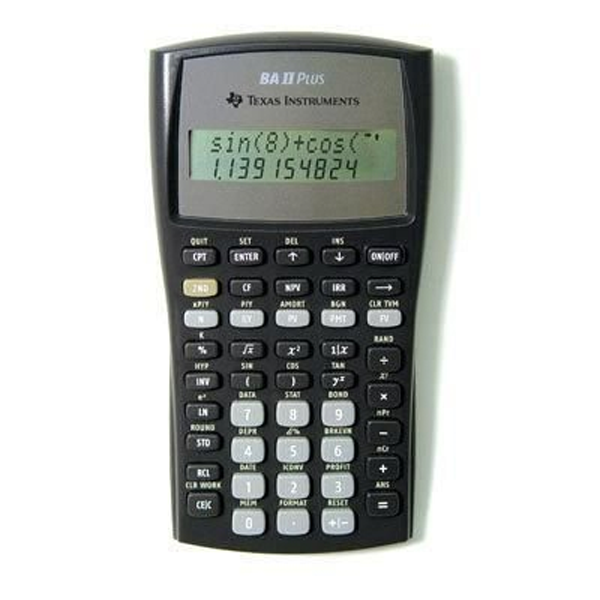# TI BA II Plus Financial CalculatorSKU:
1070346

# TI BA II Plus Financial Calculator

 \$34.95
Description:

### Key features:

• Time-Value-of-Money (TVM) calculations
• Amortization schedules
• Cash-flow analysis, Net Present Value (NPV) and Internal Rate of Return (IRR)
• Depreciation with four different methodologies
• Breakeven, profit and percent difference calculations
• Second key feature to calculate terms fast

### Exam acceptance:

• The BA II Plus is approved for use on the following professional exams:
• Chartered Financial Analyst exam
• GARP Financial Risk Manager (FRM) exam
• Certified Management Accountants exam

### Feature highlights:

• Prompted display shows current variable label and values.
• Calculate IRR and NPV for cash-flow analysis
• Store up to 24 uneven cash flows with up to four-digit frequencies; edit inputs to analyze the impact of changes in variables.
• Time-value-of-money function
• Quickly solve calculations for annuities, loans, mortgages, leases and savings.
• Depreciation schedules
• Choose from six methods for calculating depreciation, book value and remaining depreciable amount.
• Interest rate conversions
• Convert between annual (nominal) and effective interest rates.

### Ideal for:

• Accounting
• Economics
• Finance
• Marketing
• Mathematics
• Real Estate
• Science
• Statistics
• SKU:
1070346
Description:

### Key features:

• Time-Value-of-Money (TVM) calculations
• Amortization schedules
• Cash-flow analysis, Net Present Value (NPV) and Internal Rate of Return (IRR)
• Depreciation with four different methodologies
• Breakeven, profit and percent difference calculations
• Second key feature to calculate terms fast

### Exam acceptance:

• The BA II Plus is approved for use on the following professional exams:
• Chartered Financial Analyst exam
• GARP Financial Risk Manager (FRM) exam
• Certified Management Accountants exam

### Feature highlights:

• Prompted display shows current variable label and values.
• Calculate IRR and NPV for cash-flow analysis
• Store up to 24 uneven cash flows with up to four-digit frequencies; edit inputs to analyze the impact of changes in variables.
• Time-value-of-money function
• Quickly solve calculations for annuities, loans, mortgages, leases and savings.
• Depreciation schedules
• Choose from six methods for calculating depreciation, book value and remaining depreciable amount.
• Interest rate conversions
• Convert between annual (nominal) and effective interest rates.

• Accounting
• Economics
• Finance
• Marketing
• Mathematics
• Real Estate
• Science
• Statistics